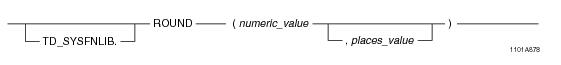# 15.00 - ROUND - Teradata Database

## Teradata Database SQL Functions, Operators, Expressions, and Predicates

Product
Release Number
15.00
Content Type
Programming Reference
Publication ID
B035-1145-015K
Language
English (United States)

## ROUND

### Purpose

Returns numeric_value rounded places_value places to the right or left of the decimal point.

### Syntaxwhere:

 Syntax element … Specifies … TD_SYSFNLIB the name of the database where the function is located. numeric_value a numeric argument. places_value the number of places to round. If not specified, numeric_value is rounded to 0 places by default.

### ANSI Compliance

This is a Teradata extension to the ANSI SQL:2011 standard.

### Invocation

ROUND is an embedded services system function. For information on activating and invoking embedded services functions, see “Embedded Services System Functions” on page 24.

### Argument Types and Rules

Expressions passed to this function must have the following data types:

• numeric_value = BYTEINT, SMALLINT, INTEGER, BIGINT, DECIMAL/NUMERIC, FLOAT/REAL/DOUBLE PRECISION, or NUMBER
• places_value = NUMBER
• For the places_value argument, you can also pass values with data types that can be converted to INTEGER using the implicit data type conversion rules that apply to UDFs. Implicit type conversion is not supported for the numeric_value argument.

Note: The UDF implicit type conversion rules are more restrictive than the implicit type conversion rules normally used by Teradata Database. If an argument cannot be converted to the required data type following the UDF implicit conversion rules, it must be explicitly cast.

For details, see “Compatible Types” in SQL External Routine Programming.

### Result Type

The result data type is the same type as that of the numeric_value argument.

If the data type of numeric_value is DECIMAL/NUMERIC with a precision less than 38, the return DECIMAL/NUMERIC value will have its precision increased by 1. For example, a DECIMAL(6,4) argument is returned as a DECIMAL(7,4). If the precision is 38, the scale will be reduced by 1 unless the scale is 0. For example, a DECIMAL(38,38) argument is returned as DECIMAL(38,37).

For information on the default data type formats, see SQL Data Types and Literals.

### Usage Notes

ROUND functions as follows:

• It rounds places_value places to the right of the decimal point if places_value is positive.
• It rounds places_value places to the left of the decimal point if places_value is negative.
• It rounds to 0 places if places_value is zero or is omitted.
• If numeric_value or places_value is NULL, the function returns NULL.
• ROUND rounds the value away from zero and it only rounds when the next digit is a value of 5 or greater.
• Note: The rounding behavior always follows the above formula regardless of the setting of the DBS Control Record RoundHalfWayMagUp field. That is, the rounding behavior always functions as if the RoundHalfWayMagUp field is set to TRUE.

### Example

The following query returns the result 32.4000.

`   SELECT ROUND(32.4467, 1);`

### Example

The following query returns the result 32.4600.

`   SELECT ROUND(32.4567, 2);`

### Example

The following query returns the result 100.0000.

`   SELECT ROUND(99.9999, 3);`

### Example

The following query returns the result 30.0000.

`   SELECT ROUND(32.4567, -1);`

### Example

The following query returns the result 100.0000.

`   SELECT ROUND(55.4567, -2);`

### Example

The following query returns the result 0.0000.

`   SELECT ROUND(55.4567, -3);`

### Example

The following query returns the result -5.00.

`   SELECT ROUND(-5.35, 0);`

### Example

The following query returns the result -6.00.

`   SELECT ROUND(-5.55, 0); `